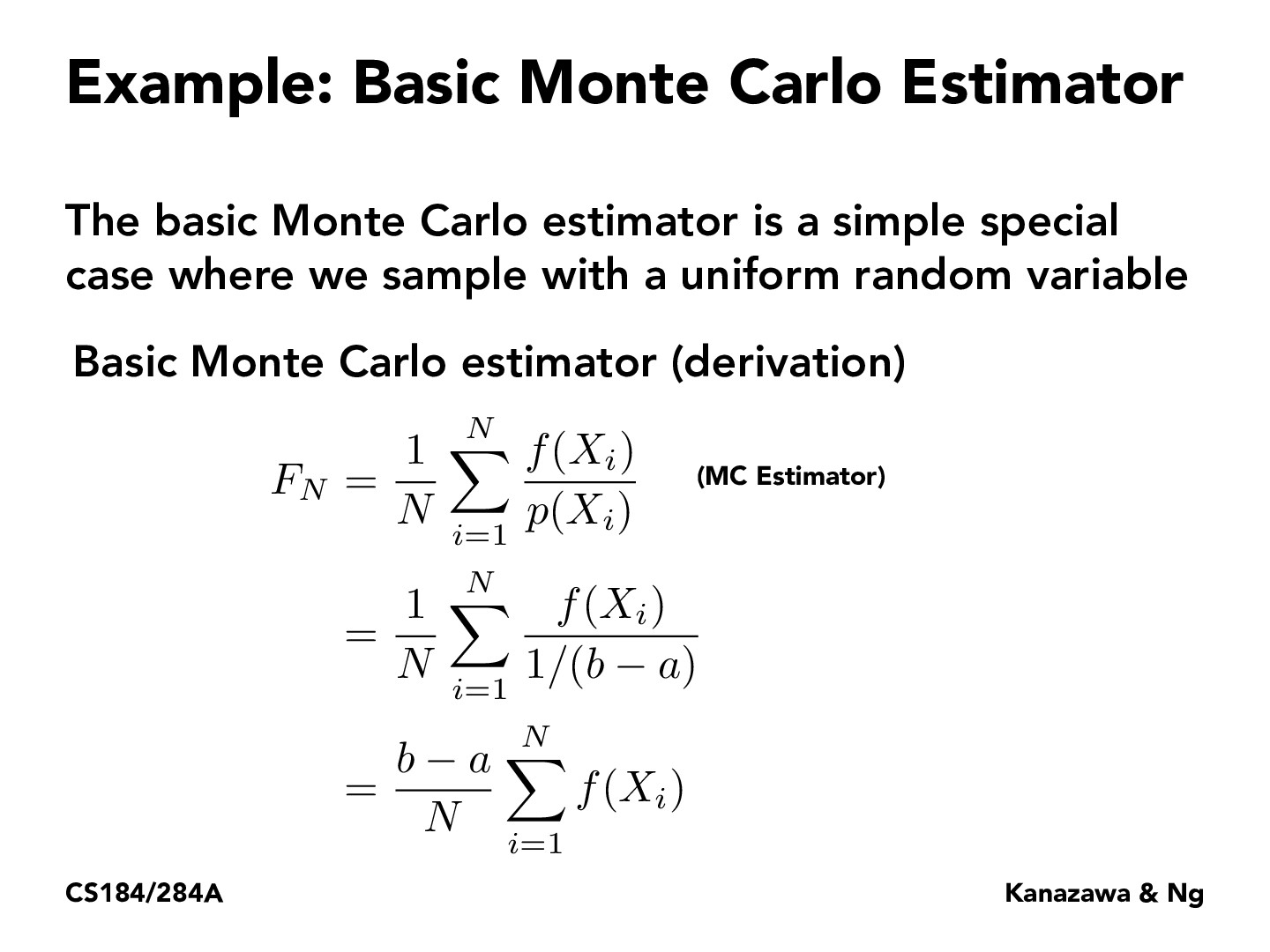Lecture 12: Monte Carlo Integration (23)YIYANGCAI

when the pdf is a constant function, it means that we are computing the integral value with range multiply the average value of the function. It means that we are finding a vertical line across the function and use the rectangle's area to represent the integral value. This is not good, I think, because we have to do many sample times to find this so-called average with an acceptable error.herojelly

I noticed we say that $p(X_i) = 1/(b-a)$. Do we always assume $X_i$ is sampled from a uniform distribution when using Monte Carlo estimators?tancik

@herojelly: We only assume $X_i$ is sampled from a uniform distribution when we say "Basic" MC estimator. Most of the time it will not be uniform.

You must be enrolled in the course to comment